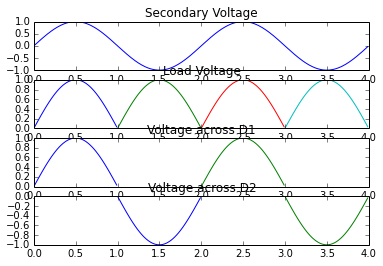# Chapter 1: Power Diodes And Transistors¶

## example 1.1, Page No. 8¶

In :
# stored charge and peak reverse current

import math
#variable declaration
t = 2.5*10**-6                # reverese recovery time to diode
di_by_dt = 35*10**6           # di/dt in A/S

#Calculations
Q= 0.5*(t**2)*di_by_dt
I= math.sqrt(2*Q*di_by_dt)

#result
print("(a) Stored charge\n Q = %.3f * 10^-6 C\n\n(b) Peak reverse current\nI = %.1f A"%(Q*10**6,I))

(a) Stored charge
Q = 109.375 * 10^-6 C

(b) Peak reverse current
I = 87.5 A


## example 1.2, Page No.8¶

In :
#Vrrm rating for diode in full wave rectifire

import math
# variable declaration
V= 12                     # secondary peak voltage
sf = 1.4                  # safety factor

#calculations
# For fullwave rectifier with transformer secondary voltage 12-0-12, each diode will experience Vrrm equal to 2 x sqrt(2)x 12
r = math.sqrt(2)
r = math.floor(r*1000)/1000
V = 2*r*V                # Actual value of Vrrm for each diode
Vrrm= V*sf

# result
print("Vrrm rating for each diode with safety factor of %.1f is %.2fV\n\n"%(sf,Vrrm))
#Answer in the book for Vrrm rating is wrong

#%pylab inline
import matplotlib.pyplot as plt
from numpy import arange,sin,pi
%matplotlib inline
#fig -1
t = arange(0.0,4,0.01)
S = sin(math.pi*t)
plt.subplot(411)
plt.title("Secondary Voltage")
plt.plot(t,S)
#fig -2
plt.subplot(412)
plt.title("Load Voltage")
t1 = arange(0.0,1,0.01)
t2 = arange(1.0,2.0,0.01)
t3 = arange(2.0,3.0,0.01)
t4 = arange(3.0,4.0,0.01)
s1 = sin((pi*t1))
s2 = sin((pi*t1))
s3 = sin(pi*t1)
s4 = sin(pi*t1)

plt.plot(t1,s1)
plt.plot(t2,s2)
plt.plot(t3,s3)
plt.plot(t4,s4)
#fig -3
plt.subplot(413)
plt.title("Voltage across D1")
plt.axis([0,4,0,1])
plt.plot(t1,s1)
plt.plot(t3,s3)
#fig -4
plt.subplot(414)
plt.title("Voltage across D2")
plt.axis([0,4,-1,0])
s2 = sin((pi*t1)-pi)
s4 = sin(pi*t1-pi)
plt.plot(t2,s2)
plt.plot(t4,s4)
#Result
plt.show()

Vrrm rating for each diode with safety factor of 1.4 is 47.51V## example 1.3, Page No. 9¶

In :
# Average, peak and rms current

import math
#variable declaration(from the waveform)
Ip = 20.0                 # Peak current

#calculations
Iavg = (Ip*1.0)/3.0
Irms = math.sqrt((Ip**2)*1.0/3.0)

#Result
print("Peak Current = %d A\nAverage Current = %.3f A\nrms Current = %.3f A"%(Ip,Iavg,Irms))

Peak Current = 20 A
Average Current = 6.667 A
rms Current = 11.547 A


## example 1.4, Page No. 18¶

In :
#power BJT

import math
# variable declaration
Vcc =220.0              # collector voltage
Vce_sat = 1.0           # Vce saturation voltage
Rb =6.0                 # base resisror
Rl = 8.0                # load resisotr
hfe = 15.0              # gain
Vbe = 0.7               # base-emiter voltage drop

#calculations
#(a)
Ic = (Vcc-Vce_sat)/Rl
Ib=Ic/hfe
#(b)
Vbb= Ib*Rb+Vbe
#(c)
Pc = Ic*Vce_sat
Pb = Ib*Vbe
Pt = Pc+Pb

#Result
print("(a) Base current, Ib = %.3f A\n(b) Vbb = %.2f V\n(c) Total power dissipation in BJT = %.4f W"%(Ib,Vbb,Pt))

(a) Base current, Ib = 1.825 A
(b) Vbb = 11.65 V
(c) Total power dissipation in BJT = 28.6525 W


## example 1.5, Page No. 18¶

In :
#Load current and losses in BJT

import math
# variable declaration(with reference to example 1.4)
Vbb_org = 11.65         # original Vbb
fall =0.85              # 85% fall in original value
Vce_sat = 1.0           # Vce saturation voltage
Rb =6.0                 # base resisror
hfe = 15.0              # gain
Vbe = 0.7               # base-emiter voltage drop

#calculations
Vbb = fall* Vbb_org
Ib = (Vbb-Vbe)/Rb
Ic = Ib*hfe
Pc =Ic*Vce_sat
Pb = Ib* Vbe
Pt = Pc+Pb

#Result
print("Load current = %.3f A\nLosses in BJT = %.2f W"%(Ib,Pt))

Load current = 1.534 A
Losses in BJT = 24.08 W


## example 1.6, Page No. 19¶

In :
# Power loss in BJT

import math
#variable declaration(with reference to example 1.4)
Vcc = 240                  # New value of collector current
Ic = 27.375                # collector current,from example 1.4
Pb = 1.2775                # base power dissipation,from example 1.4
Rl = 8.0                   # load resisotr

#Calculations
Vce = Vcc-(Ic*Rl)
Pc = Vce* Ic
Pt = Pb+ Pc

#result
print("Total power dissipation = %.4f W"%Pt)

Total power dissipation = 576.1525 W


## example 1.7, Page No. 19¶

In :
# BJT switching frequency

import math
from scipy.integrate import quad
# variable declaration
I = 80                      # maximum current, from swiching characteristics
t1 = 40 *10**-6             # rise time, from swiching characteristics
t2 = 60* 10**-6             # falll time, from swiching characteristics
V = 200                     # collector-emitter voltage
Pavg =250                   # average power loss

#calculations
# switching ON
ic = I/t1
def f(x):
return (ic*x)*(V-(V/t1)*x)
t_lower =0
t_upper = t1
val_on = quad(f,t_lower,t_upper)

# switching OFF
ic = I-I/t1
Vc = V/t2
def f1(x):
return (I-(I/t2)*x)*(Vc*x)
t_lower =0
t_upper = t2
val_off = quad(f1,t_lower,t_upper)

loss= val_on+val_off
loss= math.floor(loss*10000)/10000
f =Pavg/loss

# Result
#print("(a) Switching ON:\nEnergy losses during switching on = %.4f J"%(val_on))
#print("\n(b)Switching OFF\nEnergy losses during switching off of BJT =%.2f J"%(val_off))
print("\nSwitching frequency = %.1f Hz"%f)

Switching frequency = 937.7 Hz


## example 1.8, Page No. 20¶

In :
# Turn ON loss of power transistor

import math
#variable declaration
Vmax = 300             # voltage during start
Imax = 200             # full current after start
t = 1* 10**-6          # starting time

#calculation
E_loss = Vmax*Imax*t/6        #formula

#Result
print("Energy loss = %.2f Joules"%E_loss)

Energy loss = 0.01 Joules